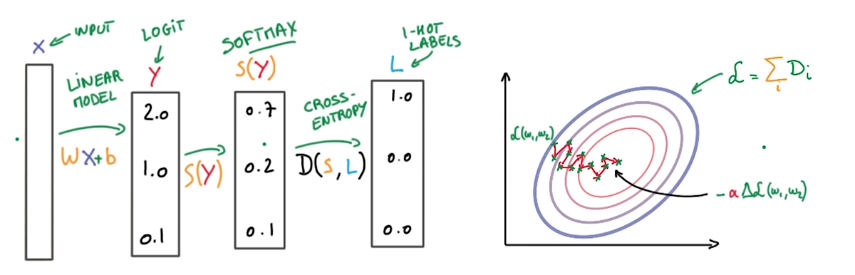# (DeepLearning MOOC) Lesson 1: From Machine Learning to Deep Learning

## Softmax function

socres `yi` ⇒ probabilities `pi`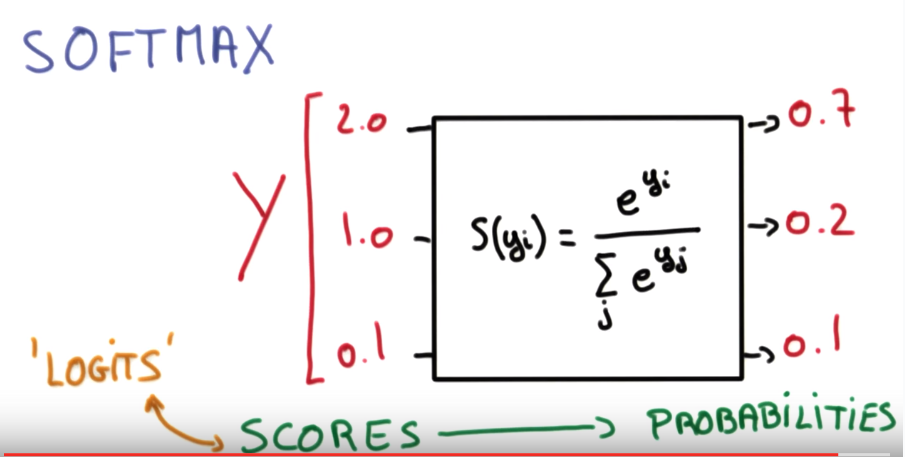property: smaller scores ⇒ less certain about result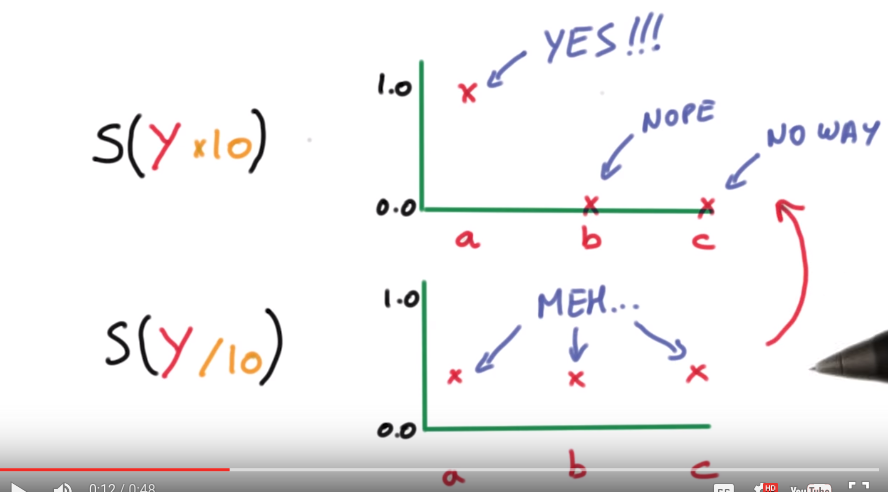## Onehot encoding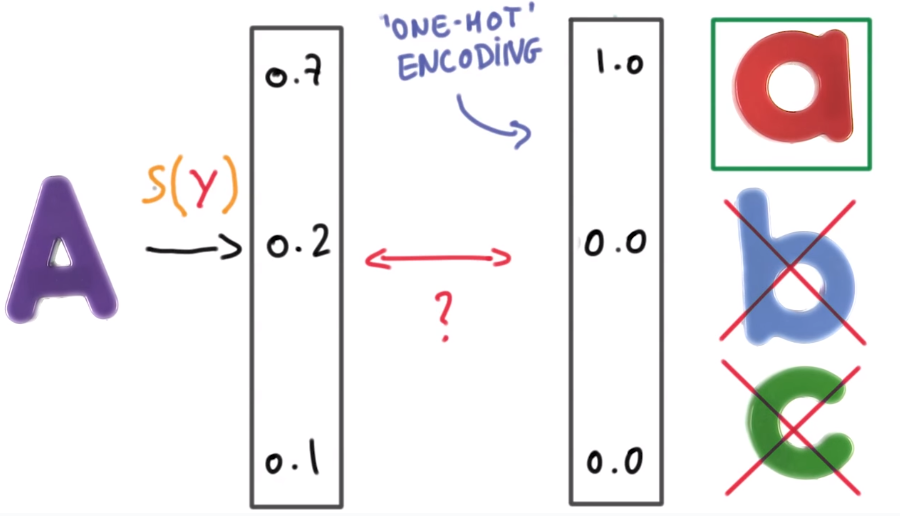## Cross entropy

measure how well the probability vector `S` corresponds to the label vector `L`. ⇒ cross entropy `D(S,L)`( D>=0, the smaller the better)N.B. `D(S,L)` is not symmetric (never log 0 )

recap ("multinominal logistic classificaton"):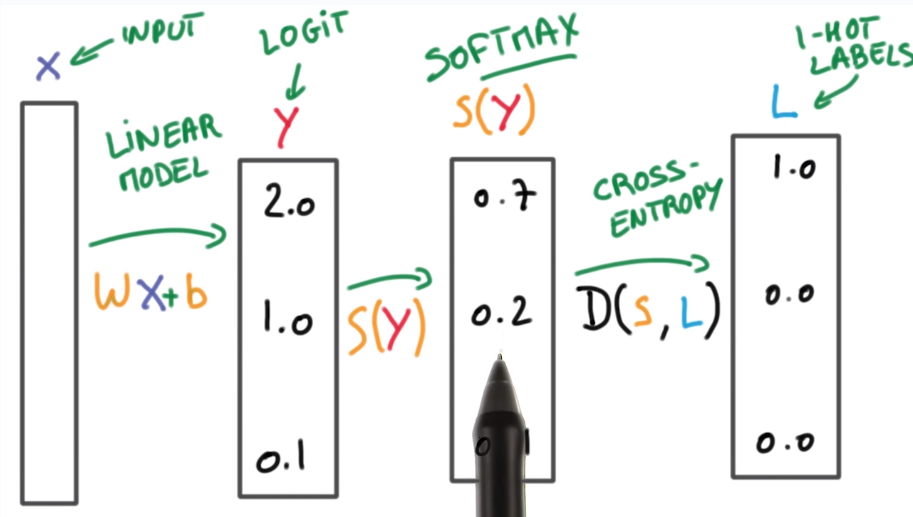## Minimizing cross entropy

take avg D as loss function:⇒ optimization, for example, by grad-desc: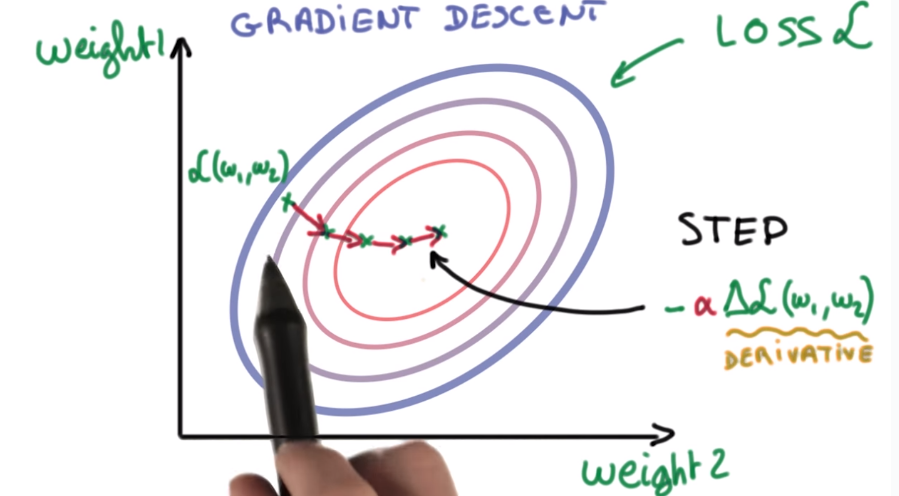for the moment, take the optimizer as black box.

two practical problems:

1. how to feed img pixels to classifiers
2. how to initialize the optimization

## numerical stability

adding very small values to very large values will introduce a lot of errors ! ex.

```>>> a = 1e9
>>> for _ in xrange(1000000):
...     a += 1e-6
>>> a - 1e9
0.95367431640625
```

⇒ the result is not 1...

⇒ normalize input ! ⇒ 0 mean, 1 variance

this make optimizers easier to find optimum.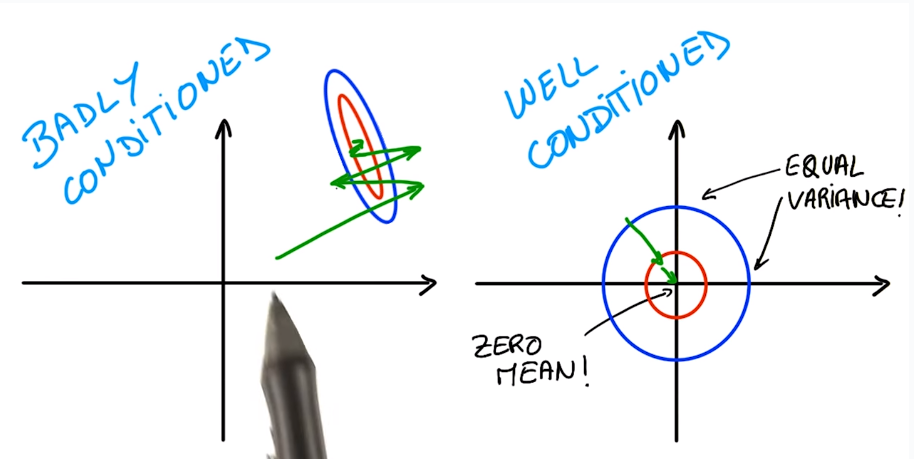normalization for images: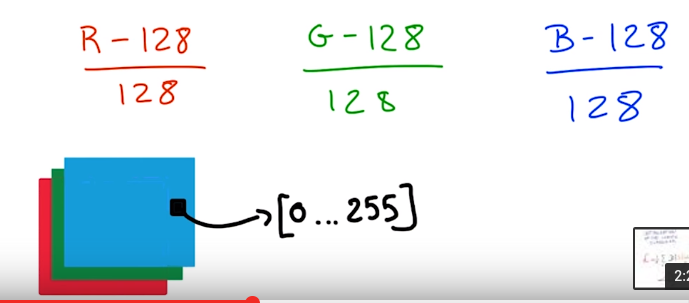## weight initialization

draw init w/b from a `Gaussian(0, sigma)`, sigma → magtitude of initial output. small sigma means small outputs → uncertain about result. ⇒ take small sigma for initializationrecap: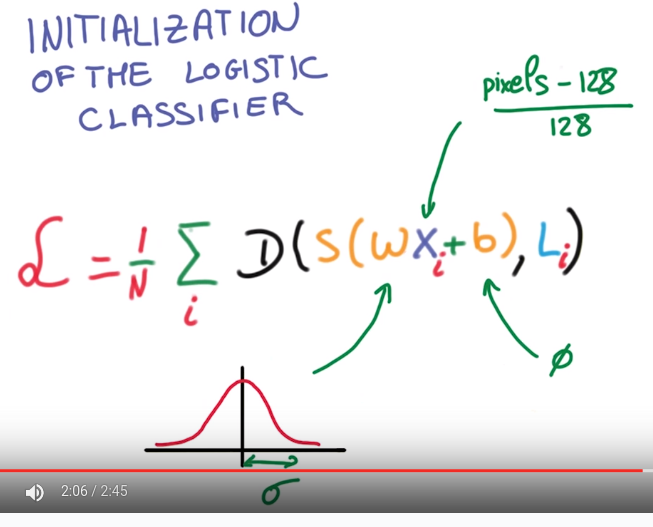⇒ feed this loss fcn to the optimizer

## training, validation and test dataset

rule of thumb (30): a change that affects 30 examples in the validation set is statically significant. ⇒ in most cases use >30000 samples in validation set → changes in 0.1% is significant.

## SGD

rule of thumb: computing `grad(L)` takes 3x time than computing loss fcn `L`. → pb for scaling..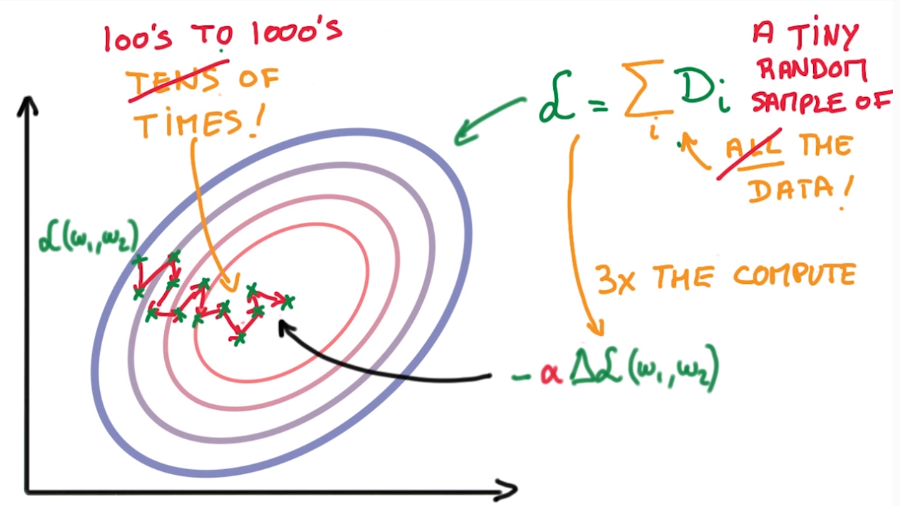SGD is the only fast enough model in practice.

tricks to help SGD:

1. normalize data (0 mean, uni-var)
2. randomly initialize weights
3. momentum
4. learning rate decay

## Momentum

SGD: many small steps in random directions → general direction is more accurate. ⇒ keep a running average of the gradients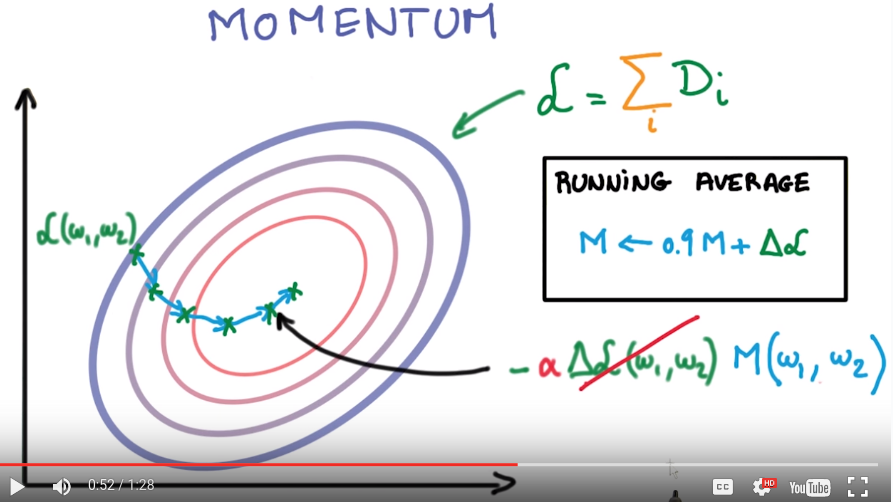## Learning rate decay

take smaller and smaller steps (alpha decays) e.g. alpha decays exponentially...## parameter tuning

how quickly you learning != how well you train..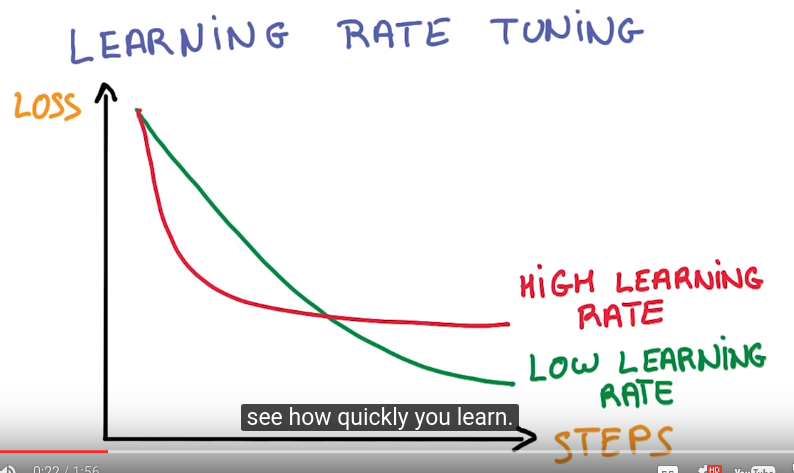balck magics in deep learning: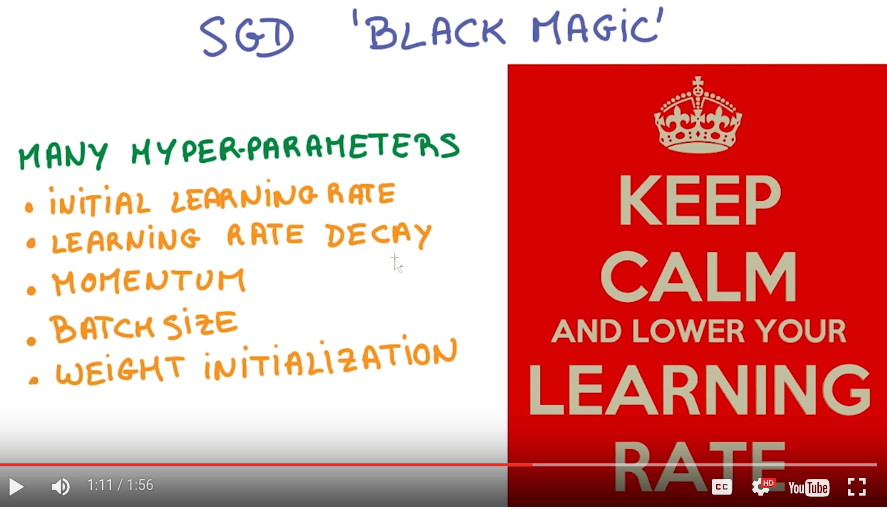recap: